# Resonance in parallel RL-RC circuit

• Engineering

## Homework Statement

calculate R2, so the resonance is still posible ?
C = 40 μF
R1 = 30 ohm
L = 9mH

image : http://postimg.org/image/kampvczv5/

## Homework Equations

ω = 1/sqroot(L*C)
c = 1/(ω*C)
XL = ω * L
Z = (Z1*Z2)/(Z1+Z2)

## The Attempt at a Solution

My approach was to first calculate the resonance frequency ω = 1/sqroot(L*C) = 1667 s-1 than
XL = ω * L ; Xc = 1/(ω*C) == 15ohm, since they have to be the same to cancel out if I'm correct.
And at last got the R2 = 30ohm using the equation Z = (Z1*Z2)/(Z1+Z2) but the right answer should be R2 > 15ohm, so I don't know how to tackle this problem and I'm asking you guys for some advice/solution.

BvU
Homework Helper
Hello Kamen,Do us a favor and don't post pictures on sites where one gets smothered with pictures of hookers by russian criminals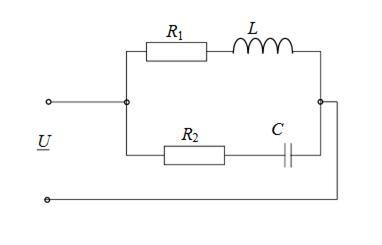Did you render the complete problem statement ?

Did you already learn to work with complex impedances ? It seems to me that's required here....

What is the criterion for resonance ? How do you find the resonance frequency when there are resistors present ?

My approach was to first calculate the resonance frequency ω = 1/sqroot(L*C) = 1667 s-1
No - you cannot (blindly) assume that the resonant frequency is the same as for a lossless tank circuit.
.... since they have to be the same to cancel out if I'm correct.
Yes - that is the approach pointing into the right direction:
In words: At resonance, both imaginary parts of the resulting impedance (or admittance) must cancel each other.
That means: You are looking for the frequency at which the resulting input impedance of the circuit is REAL.
Therefore: Find the expression for the total admittance Y(jw) at the input (this is simpler than finding the impedance Z(s)=1/Y(s) ) and split it into real and imag. parts.
Finally, set Im(Y)=0 and solve for w.
This expression gives you the resonant frequency as a function of all 4 elements.

•Kamen12
Thank you BvU for the welcome and sorry for the hooker ads, didn't know how to post a picture :)
Thanks for the advice "LvW" I used the admittance this time and set the im(Y) = 0 just like you said and I solved the problem, I will post the picture of the problem solved for anyone looking for the solution.

Anyone looking for the basic equations used in this problem : https://en.wikipedia.org/wiki/Admittance
HQ picture of solution : https://mega.nz/#!tJ0mXKII!qWFYuqfMc8Bsr1RLLWfeaWbTHXtF_LeVgjp77pMnUE4

#### Attachments

BvU
Homework Helper
Bravo !The Electrician
Gold Member

Given these values:
C = 40 μF
R1 = 30 ohm
L = 9mH

What do you calculate for a frequency of resonance? What is the value of the imaginary part of the admittance at that frequency?

Given these values:
C = 40 μF
R1 = 30 ohm
L = 9mH

What do you calculate for a frequency of resonance? What is the value of the imaginary part of the admittance at that frequency?

Sorry, but forgot to mention the results in my previous post, here are my "findings":

After I've gotten the result that R2 > 15

1) R2 = 10 ohm
i took that R2 = 10ohm than inserted that into the equation for
angular frequency (w), and found out I get negative number in the
denominator and positive in numerator which gives me a negative number under a square root function.
1) R2 = 10 000 ohm
R2 = 10 000 ----> angular frequency (w) equation
got angular frequency (w) = 790,57s-1
and than inserting that into equation for imaginary part of the admittance I got a value of around
0 (approximation error)

Sorry, but forgot to mention the results in my previous post, here are my "findings":
Here are my results:
After setting Im(Y)=0 we get an expression for the frequency w.
This expression is a function consisting of a numerator N and a denominator D.
Both must be positive for a positive frequency (N>0, D>0).
From this we get:
a) (R1)²>L/C=225 (V/A)² and
b) (R2)²>L/C=225 (V/A)².

R1=30 ohms can fullfill equation a) and for R2 we find R2>SQRT(225)=15 ohms.

The Electrician
Gold Member
I derive an expression for the impedance of the circuit like this: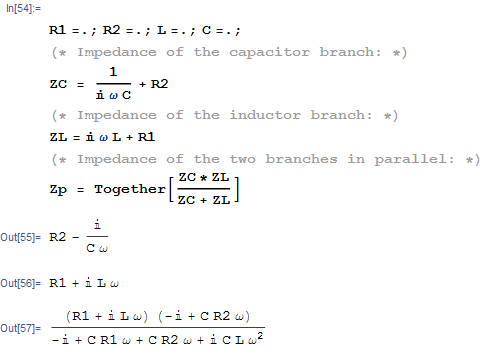Now, for a sanity check, I plot over a wide frequency range my expression for the imaginary part of Z (blue) and Kamen12's expression for the imaginary part of the admittance (red) for values of R1=5 and R2 = 5. I see plainly that the imaginary parts do become zero at a certain radian frequency: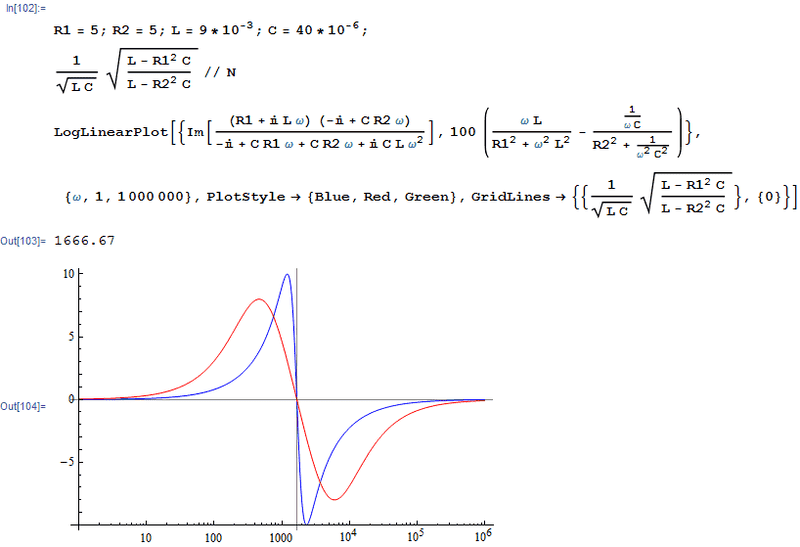Now, let R1 become 30 ohms and let R2 remain at 5 ohms. A plot shows that the imaginary part is never zero, even though R2 is only 5 ohms. When R2 is 15 ohms, the situation is even worse; the imaginary part is never zero, so we have no resonance: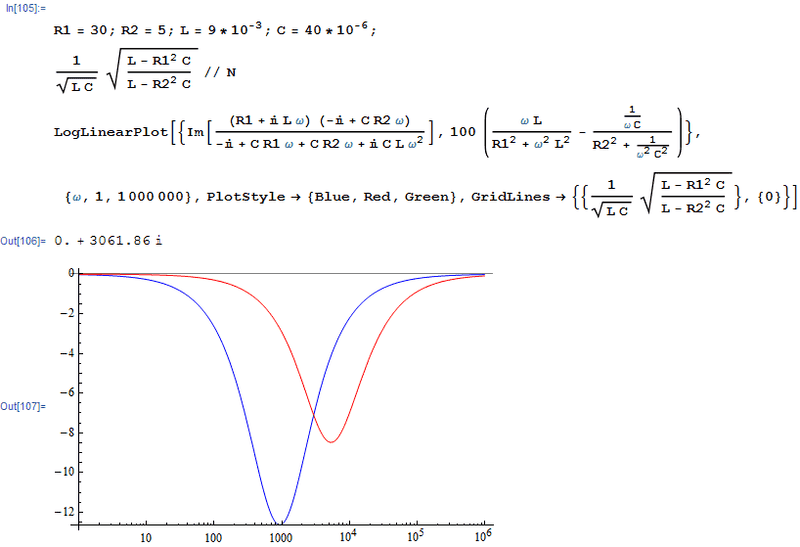But, if for example, we make C = 4 uF rather than 40 uF, with R1=30 and R2=15 we do get a resonance: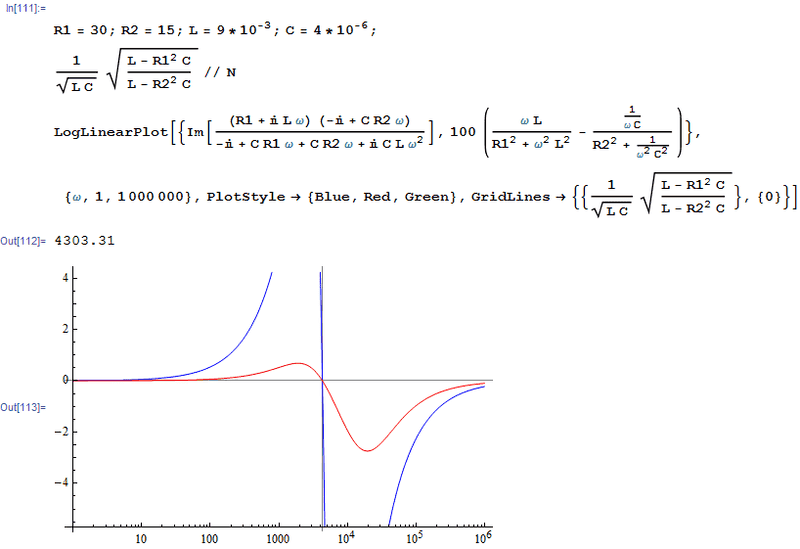It appears that with the given values for R1, L and C, there is no value of R2 which will give a resonance.